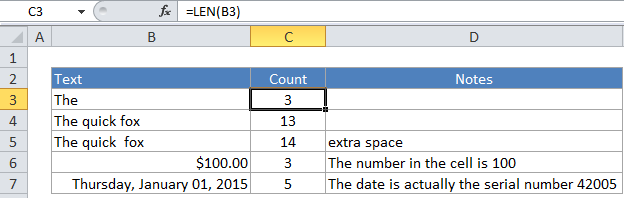## Excel Office

Excel How Tos, Tutorials, Tips & Tricks, Shortcuts

# How to count number of characters of text in a cell in Excel

If you need to count the total characters in a cell, you can do so using the LEN function.

## Formula

`=LEN(a1)`## Explanation

In the example, the formula in the active cell is:

`=LEN(B3)`

The LEN function simply counts all characters that appear in a cell. All characters are counted, including space characters. Numbers are also counted in their raw form (i.e. number formatting is not included).

Also See:   How to count total words in a cell in Excel